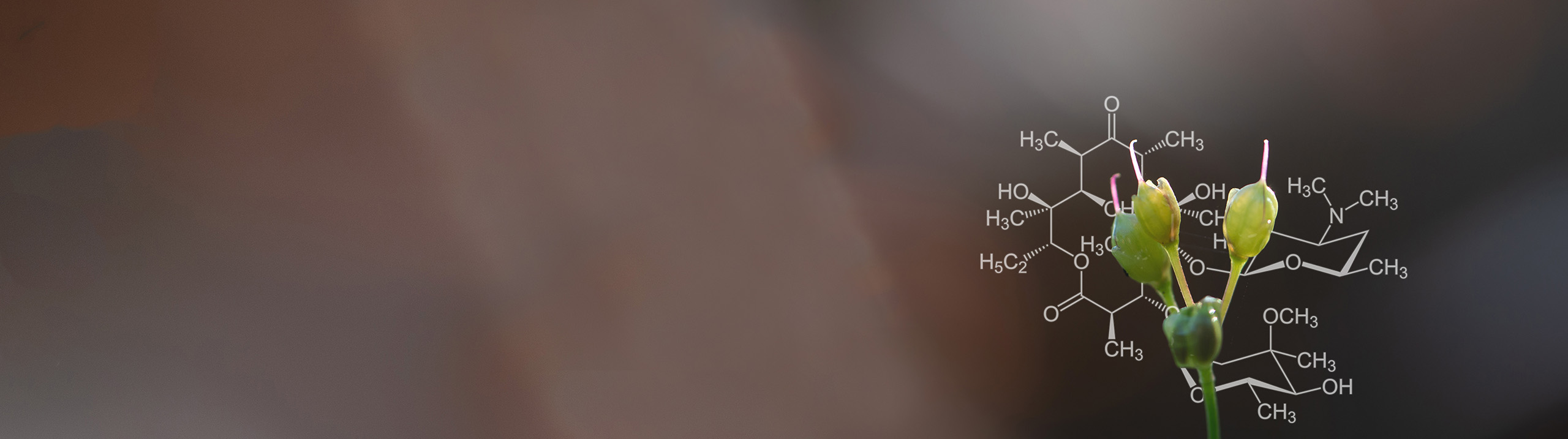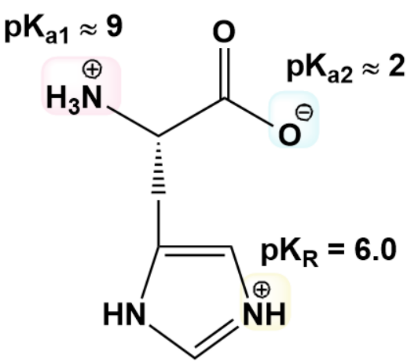Start typing, then use the up and down aroows to select an option from the list.# Biochemistry

Learn the toughest concepts covered in Biochemistry with step-by-step video tutorials and practice problems by world-class tutors

3. Amino Acids

# Amino Acids and Henderson-Hasselbalch

1

### concept

Amino Acids and the Henderson-Hasselbalch Equation2m
Play a video:
0
2

### example

Amino Acids and Henderson-Hasselbalch4m
Play a video:
1
3
Problem

At pH 11.8, what is the % of protonated amino group in the R-group of Lysine. (Lysine’s pKR = 10.8)?

4
Problem

Draw Glu & calculate the % of -COOH in the R-group of Glu at pH 3.2. (Glu pK  R = 4.1).

5
Problem

Draw Asp & calculate the pH at which two thirds (2/3) of Asp’s R-group is dissociated. (Asp pKR = 3.9).

6
Problem

Draw Arg & calculate the pH at which 23% of Arg’s R-group is dissociated. (Arg pK  R = 12.5).

7
Problem

What is the ratio of [conjugate base] to [conjugate acid] for each of Histidine’s three ionizable groups at pH 7?A) Amino group ratio: ________________

B) Carboxyl group ratio: ________________

C) R-group ratio: ________________

D) Use the ratios above to determine the average net charge of the ionizable groups & the entire His molecule.

1. Net charge of Amino groups: ____________

2. Net charge of Carboxyl groups: ____________

3. Net charge of R-groups: ____________

4. Net charge of His: ____________ (Hint: sum previous 3 charges).